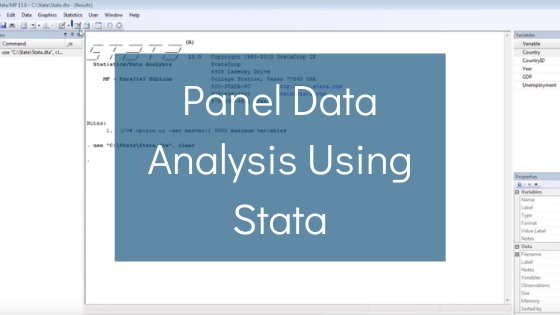Phone: (+44) 0203 9500 830

Whatsapp: (+44) 0794 0000 629

# Panel Data Analysis Using StataTo conduct a panel regression analysis in Stata, the following steps should be done. First, a panel dataset should be uploaded into Stata using the command

where excel is the software in which the dataset is created, and firstrow is the command that lets Stata store the first row as variable names.

Next, the data should be recognised as panel data by the software. This is done using the following command

## xtset var1 var2

where var1 and var2 are the identifiers for the time period and cross-section. Normally, these variables are the time variable such as year, month or day, and a cross-section ID such as country, individual, or company.

The next step of the analysis is the assessment of descriptive statistics using the command

## summarize var1 var2 … varn

where var1varn are the explored variables.

It is also useful to test whether there is multicollinearity in the data sample. Multicollinearity is the phenomenon when independent variables are strongly correlated. In this case, the outcomes of the regression analysis may be inaccurate since it will be difficult to estimate the individual effects of explanatory variables on the dependent variable. The table of pairwise correlations is formed using the command

## pwcorr var1, var2 … varn

where var1 … varn are all the independent variables in the model.

Next, the panel regression is run using the command

## xtreg depvar var1 … varn

where depvar is the dependent variable and var1… varn are all the explanatory variables.

It is also necessary to distinguish between fixed effects (FE) and random effects (RE) in the model. When conducting a panel regression analysis, it is important to account for time-specific differences such as abnormal or crisis years and cross-sectional differences such as unique features of companies or countries investigated. Such features are usually not captured by the explanatory variables, and therefore, there are two options to deal with this problem. The first option is to add dummy variables for periods or cross-sections. This is what is known as the FE specification. An alternative option is to introduce a new stochastic variable that would account for cross-sectional or time variability. This is what is known as the RE specification. In the latter case, a new error term is added to the model, which could be a cause of inconsistent coefficients.

To test whether the RE-model is consistent, the Hausman specification test is conducted. To do this, the model is first run with the FE specification using the command

## xtreg depvar var1 … varn, fe

and storing the results using the command

## estimates store fe1

where fe1 is the name of the new variable which contains the stored results.

The same procedure is then conducted using the RE specification.

After that, the Hausman test is conducted by running the following command

## hausman fe1 re1

where fe1 and re1 are the stored FE and RE values, respectively. The null hypothesis of the test is that the coefficients of the RE-model are consistent. If the null hypothesis cannot be rejected, then the RE-model results are used in the analysis. In the opposite case, those of the FE-model are employed.

Next up: Watch our handy STATA panel regression analysis tutorial over on our YouTube channel.